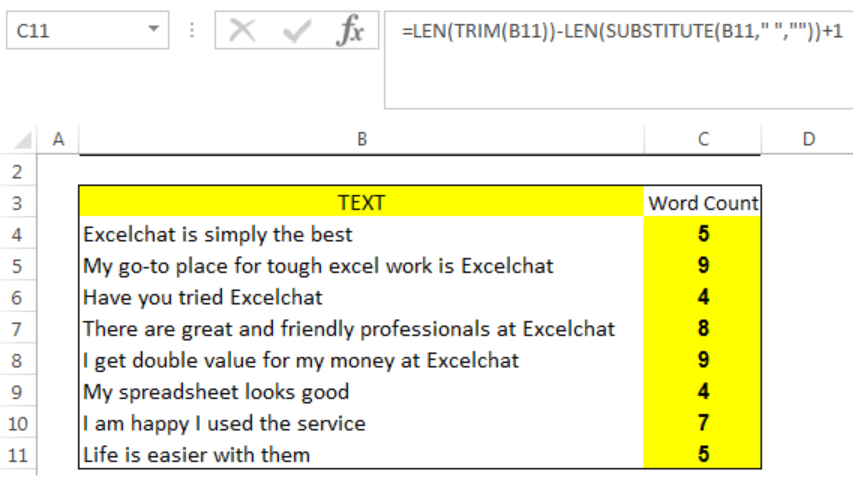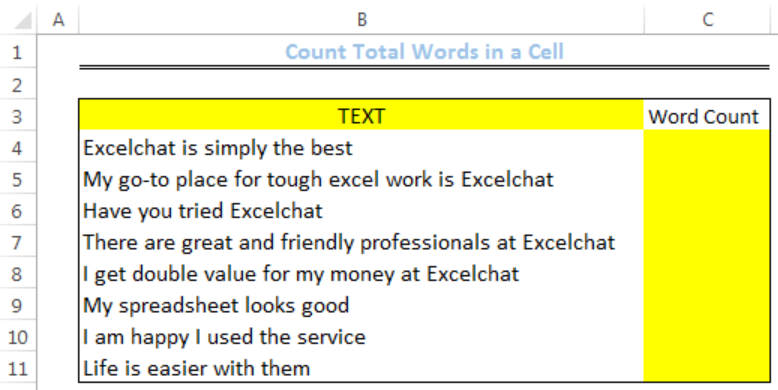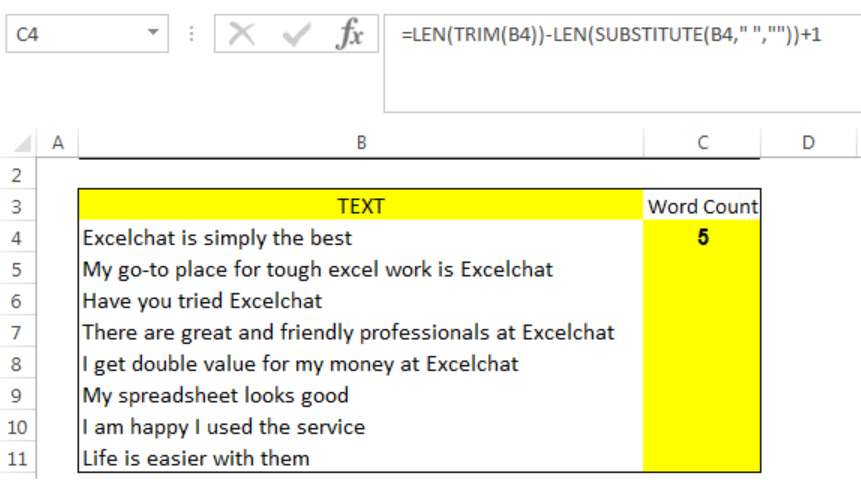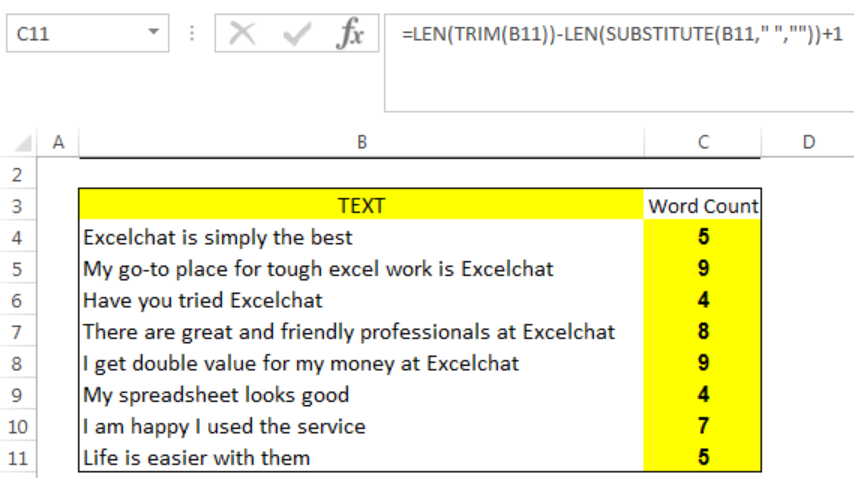Get instant live expert help with Excel or Google Sheets“My Excelchat expert helped me in less than 20 minutes, saving me what would have been 5 hours of work!”

#### Post your problem and you'll get expert help in seconds

Your message must be at least 40 characters
Our professional experts are available now. Your privacy is guaranteed.

# How to Count Total Words in a Cell

We can use a formula that combines the LEN, TRIM, and SUBSTITUTE functions to count the total words in a cell. The steps below will walk through the process.Figure 1: Count of Total Words in Cells of Column B

## General Formula

`=LEN(TRIM(A1))-LEN(SUBSTITUTE(A1," ",""))+1`

• A1 represents the cell of interest.

## Formula

`=LEN(TRIM(B4))-LEN(SUBSTITUTE(B4," ",""))+1`

## Setting up the Data

• We will set up the data by inputting the TEXT into Column B
• Column C is where we want the formula to return the result for the word count of the TEXT in Column BFigure 2: Setting up the Data

## Count Total Words in a Cell

• We will click on Cell C4
• We will insert the formula below into the cell
`=LEN(TRIM(B4))-LEN(SUBSTITUTE(B4," ",""))+1`
• We will press the enter keyFigure 3: Count of Total Words in Cell B4

• We will click on Cell C4 again
• We will double-click on the fill handle (the small plus sign at the bottom right of Cell C4) and drag down to copy the formula into the other cellsFigure 4: Count of Total Words in Cells of Column B

## Explanation

`=LEN(TRIM(B4))-LEN(SUBSTITUTE(B4," ",""))+1`

The SUBSTITUTE function gets rid of all spaces from the text. The LEN function calculates the length of the text without spaces. The total count of words in each cell is calculated by subtracting the count of the text without space from the count of the text with space. 1 is added to the result because the total number of words is equal to the number of spaces in the text plus 1.

The TRIM function gets rid of all extra spaces before, within, and after the text. However, if the cell is empty, the formula returns 1 and not zero. To prevent such issues, we will introduce IF and ISBLANK into the formula.

`=IF(ISBLANK(A1),0,LEN(TRIM(A1))-LEN(SUBSTITUTE(A1," ",""))+1`

## Instant Connection to an Expert through our Excelchat Service

Most of the time, the problem you will need to solve will be more complex than a simple application of a formula or function. If you want to save hours of research and frustration, try our live Excelchat service! Our Excel Experts are available 24/7 to answer any Excel question you may have. We guarantee a connection within 30 seconds and a customized solution within 20 minutes.

### Did this post not answer your question? Get a solution from connecting with the expert.Another blog reader asked this question today on Excelchat:## Subscribe to Excelchat.coAnother blog reader asked this question today on Excelchat: